• Call Now

1800-102-2727•

# Difference Between Exothermic and Endothermic Reactions-Introduction, Energy Diagrams, Differences, Practice Problems, FAQs

It's a cold winter night and a man is all clad in a jacket and warming up himself in front of a fireplace.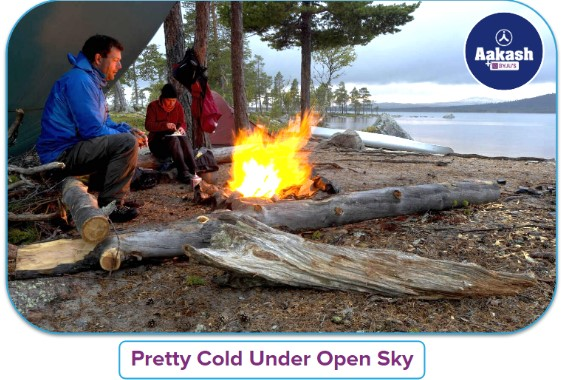It’s the night that this hiker has to spend under an open sky and it is going to be pretty cold out there. But this fire here will surely keep him warm. What do you think would be happening while he is sitting next to the fire and absorbing all the heat? But how do you look at this process from a thermodynamic point of view? Do you think he is only absorbing the heat energy or do you think that he is also emitting some energy in the form of heat as well? Let’s look at two types of processes/reactions in detail which occur in nature where heat energy is either absorbed or released.

• Introduction to Exothermic and Endothermic Reactions
• Energy Diagram of Exothermic and Endothermic reactions
• Difference Between Endothermic and Exothermic Reactions
• Identification of Exothermic and Endothermic Reactions
• Practice Problems
• Frequently Asked Questions – FAQs

## Introduction to Exothermic and Endothermic Reactions

Consider what is happening to the wood that is burning and emitting heat. This means that the combustion process generates heat, and the burning process is referred to as an exothermic process in thermodynamics. Exo means "outside," and ‘thermo’ means "heat." As a result, the term exothermic refers to a process that releases heat or emits heat into the environment.

But, if we consider this man near the fireplace as the system, he is absorbing heat, which is known as an endothermic process in thermodynamics. Because "endo" stands for internal, the term endothermic refers to a process that absorbs heat.

As a result, chemical processes or reactions are broadly classified into two types: exothermic reactions and endothermic reactions.

Exothermic Reactions

The exothermic reaction is defined as a reaction where the system (where the reaction is occurring) releases the energy to the surrounding in the form of heat. A few examples are neutralisation reactions, burning of substances, burning of fuels, deposition of dry ice, respiration, dissolution of sulphuric acid into water etc.

The term ‘Exo’ refers to ‘to release outside’ and ‘thermic’ refers to ‘heat.’

Endothermic Reactions

Endothermic reactions absorb heat energy from their surroundings. An exothermic reaction, in contrast, liberates heat energy from the system into the surrounding environment.

The endothermic reaction is defined as a reaction where the system (where the reaction is occurring) absorbs the energy from its surrounding in the form of heat. A few examples of the endothermic process are photosynthesis, evaporating liquids, melting of ice, vapourisation of dry ice, cracking of alkanes, thermal decomposition, ammonium chloride in water and much more.

## Energy Diagram of Exothermic and Endothermic reactions

Let’s consider a reaction:

A + B → C + D; ΔH = +ve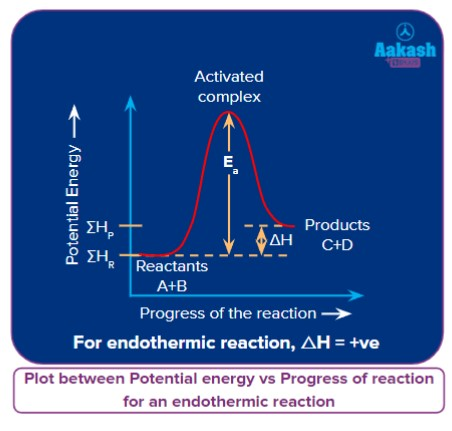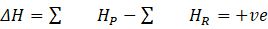for an endothermic reaction.

i.e.,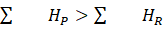Let’s consider a reaction:

A + B → C + D; ΔH= -ve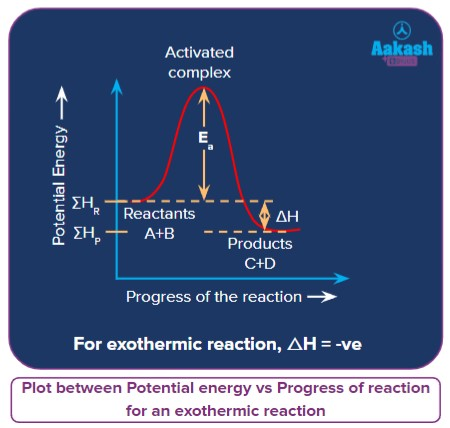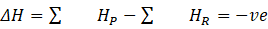for an exothermic reaction.

i.e.,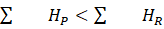The heat energy is produced as a result of the reaction happening where reactants are converted into products. It occurs as a result of the dissociation of the bonds between the molecules. The heat energy is then released through the formation of new bonds.

## Identification of Exothermic and Endothermic Reactions

Procedure to differentiate between exothermic and endothermic reactions is to either calculate theoretically the enthalpy differences between the products and reactants of the reaction or to experimentally measure the temperature of the reaction.

By Measurement of Temperature:

The temperature of the reaction can be measured before and during the reaction. An increase in temperature indicates an exothermic reaction and a lowering of temperature indicates endothermic reactions.

Calculation of Enthalpy change of a reaction (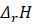)

The enthalpy change of a reaction is the enthalpy(energy) changes that take place when reactants are converted into products in a specific chemical reaction at any temperature and pressure. It is signified and determined as follows:

For example, consider the reaction given,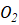(g) +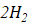(g) → 2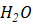(g)= (Sum of enthalpies of products) – (Sum of enthalpies of reactants)= 2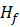(, g) – [(, g) + 2(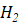, g)]

Where,= Enthalpy of formation of water

It can be calculated from,= Sum of the energy changes in reactants + Sum of the energy changes in products

In the reaction, we need two hydrogen atoms by breaking the hydrogen molecule to form water requiring energy equivalent to the binding energy of hydrogen which is 433kJ.

We need also one oxygen atom and hence the energy of half of the binding energy to break the oxygen molecule equivalent to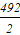kJ = 246kJ

In water, two O-H bonds are formed by releasing the binding energy of the bonds, which is equivalent to 2x464kJ.= (433 +246) +( - 928) = - 249kJ,

There is a net release of energy and hence this is an exothermic reaction.

The values of the enthalpies are added to the above equation because, according to the description, the energy used in breaking the bonds of a reactant tends to be positive (+) and the energy used in making the bonds of products tends to be negative (-).

When the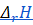is negative, a reaction is exothermic because the energy released to form the products is much greater than the energy used to break the bonds of the reactants.

When theis positive, a reaction is endothermic because the energy released to make the products is much less than the energy absorbed to break the bonds of the reactants.

Practice Problems

Q1. When energy released to form the products is much greater than the energy used to break the bonds of the reactants, the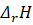should be

a. Negative
b. Positive
c. Zero
d. None of these

This is the case of exothermic reactions where the energy released during the formation of product is much greater than the energy required to break the bonds of reactant. According to the description, the energy used in breaking the bonds of a reactant tends to be positive (+) and the energy used in making the bonds of products tends to be negative (-).

Hence,should be negative and the correct answer is option (A).

Q2. Which of the following describes an endothermic reaction?

a. Formation of ice
b. Boiling of water
c. Combustion LPG in gas cylinder
d. None of the above

The boiling of water is the process, where water boils into the vapours at its boiling point i.e., 100 ℃. This boiling process happens when the water absorbs the heat from the surrounding. When the heat is absorbed, the force that holds the molecules together weakens up and liquid water is converted into vapour form, the process of absorbing heat from the surroundings is known as the endothermic process. In all other options, heat energy is being released making them exothermic processes.

Q3. When energy released to form the products is much less than the energy used to break the bonds of the reactants, theshould be

a. Negative
b. Positive
c. Zero
d. None of these

This is the case of endothermic reactions where the energy released during the formation of product is much lesser than the energy required to break the bonds of reactant. According to the description, the energy used in breaking the bonds of a reactant tends to be positive (+) and the energy used in making the bonds of products tends to be negative (-).

Hence,should be positive and the correct answer is option (B).

Q4. Which of the following describes an exothermic reaction?

a. Melting of ice
b. Sublimation of solid to gas
c. Formation of ice
d. None of these

When water is placed in a freezer, it gradually loses heat to the cold air around it. When water molecules lose energy, they move slowly, get closer, and pack together tightly enough to form ice. This is an exothermic process because the water releases heat into the surroundings.

Hence, the correct answer is option (C).

## Frequently Asked Questions – FAQs

Q1. In a chemical reaction, why is heat released or absorbed?
Answer: Chemical bonds are either broken or formed in any chemical reaction. Heat is released when chemical bonds are formed, and heat is absorbed when chemical bonds are broken. Since molecules want to remain together, forming chemical bonds between them requires less energy than breaking them, resulting in heat being absorbed from the surroundings.

Q2. Define the enthalpy of a reaction.
Answer: The enthalpy of a reaction is defined as the heat energy change that occurs when reactants become products. ΔH is positive if heat is absorbed during the reaction and if heat is released, ΔH is negative.

Q3.During the endothermic reaction, what type of energy conversion will take place?
Answer: This is the conversion of kinetic energy to chemical energy. Heat is absorbed and converted into chemical energy during an endothermic reaction.

Q4. Why is respiration regarded as an exothermic reaction?
Answer: We all know respiration involves taking up oxygen and giving up carbon dioxide. The oxygen is used up in the cell for burning glucose molecules in them to produce the energy needed for them, which is an exothermic process. Carbon dioxide is one of the products of burning. Since respiration involves an exothermic reaction of its oxygen, we say respiration is an exothermic process.

Q5.What is the main difference between an exothermic and an endothermic process?
Answer: The main difference between exothermic and endothermic reactions is that an endothermic reaction absorbs energy in the form of heat from the surroundings, whereas an exothermic reaction releases energy to the surroundings.

Related Topics:Talk to our expert
Resend OTP Timer =
By submitting up, I agree to receive all the Whatsapp communication on my registered number and Aakash terms and conditions and privacy policy